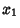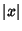## Symmetric Function

A symmetric function onvariables, ...,is a function that is unchanged by any Permutation of its variables. In most contexts, the term ``symmetric function'' refers to a polynomial onvariables with this feature (more properly called a ``symmetric polynomial''). Another type of symmetric functions is symmetric rational functions, which are the Rational Functions that are unchanged by Permutation of variables.

The symmetric polynomials (respectively, symmetric rational functions) can be expressed as polynomials (respectively, rational functions) in the Elementary Symmetric Functions. This is called the Fundamental Theorem of Symmetric Functions.

A functionis sometimes said to be symmetric about the y-Axis if. Examples of such functions include(the Absolute Value) and(the Parabola).

See also Elementary Symmetric Function, Fundamental Theorem of Symmetric Functions, Rational Function

References

Macdonald, I. G. Symmetric Functions and Hall Polynomials, 2nd ed. Oxford, England: Oxford University Press, 1995.

Macdonald, I. G. Symmetric Functions and Orthogonal Polynomials. Providence, RI: Amer. Math. Soc., 1997.

Petkovsek, M.; Wilf, H. S.; and Zeilberger, D. ``Symmetric Function Identities.'' §1.7 in A=B. Wellesley, MA: A. K. Peters, pp. 12-13, 1996.